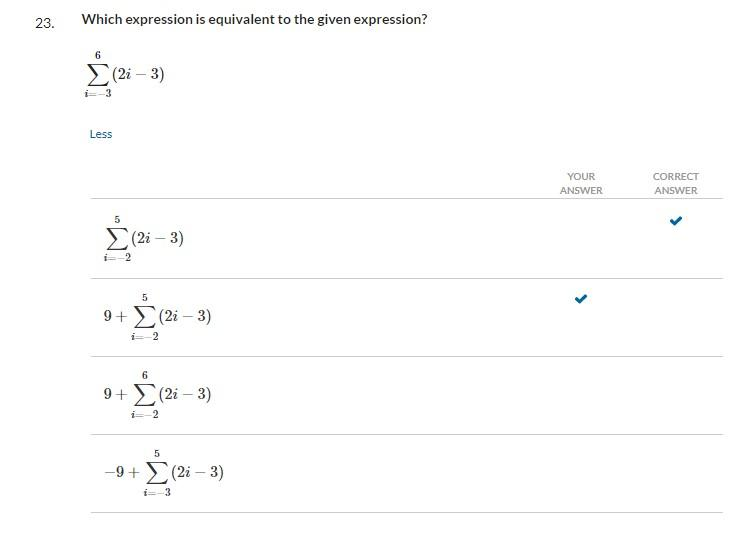Home / Expert Answers / Other Math / 23-which-expression-is-equivalent-to-the-given-expression-sum-i-3-6-2-i-3-less-pa944

# (Solved): 23. Which expression is equivalent to the given expression? $\sum_{i=-3}^{6}(2 i-3)$ Less $\ ...23. Which expression is equivalent to the given expression? \[ \sum_{i=-3}^{6}(2 i-3)$ Less $\sum_{i=-2}^{5}(2 i-3)$ $\begin{array}{l} 9+\sum_{i=-2}^{5}(2 i-3) \\ 9+\sum_{i=-2}^{6}(2 i-3) \end{array}$ $-9+\sum_{i=-3}^{5}(2 i-3)$

We have an Answer from Expert